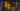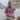# JavaScript Addition Operator in Details

Posted January 2, 2016Start discussion

## Introduction

JavaScript is an awesome language. I like it because of the flexibility: just do the things in a way you like: change the variable type, add methods or properties to object on the fly, use operators on different variable types and much more.

However the dynamism comes with a price. Developer needs to understand how to handle types conversion for different operators: addition (`+`), equality (`==` and `===`), inequality (`!=` and `!==`), etc. Many operators have their own way to handle the type conversions.

One of the most commonly used operator is addition: `+`. This operator is used to concatenate strings or sum the numbers:

1. Strings concatenation:

``````var result = "Hello, " + "World!";
// string + string = string (concatenation)
// "Hello, World!"``````

``````var result = 10 + 5;
// number + number = number (addition)
// 15``````

JavaScript allows to use objects, arrays, `null` or `undefined` as operands. Let’s try to demystify the general rule of conversion.

## Conversion rules

Use following scheme to see how JavaScript converts types in addition operation:

``operand + operand = result``
1. If at least one operand is an object, it is converted to a primitive value (string, number or boolean);
2. After conversion, if at least one operand is string type, the second operand is converted to string and the concatenation is executed;
3. In other case both operands are converted to numbers and arithmetic addition is executed.

If both operands are primitive types, then operator checks if at least one is string and executes the concatenation. In other case it just transforms everything to numbers and sum.

## Object to primitive

The object to primitive conversion:

• If object type is Date, then `toString()` method is used;
• In other case `valueOf()` method is used, if it returns a primitive value;
• In other case (if `valueOf()` doesn’t exist or doesn’t return a primitive value), then `toString()` method is used. This happens most of the times.

When an array is converted to a primitive, JavaScript uses its `join(',')` method., e.g. the primitive of `[1, 5, 6]` is `"1,5,6"`. The primitive value of a plain JavaScript object `{}` is `"[object Object]"`.

## Learning from examples

The following examples help to understand the simple and complex cases of the conversions.

Example 1: Number and string

``var result = 1 + "5"; // "15"``

Explanation:

1. `1 + "5"` (The second operand is a string and based on rule 2 the number `1` becomes `"1"`)
2. `"1" + "5"` (Strings concatenation)
3. `"15"`

The second operand is a string. The first operand is converted from number to string and the concatenation is done.

Example 2: Number and array

``var result = [1, 3, 5] + 1; //"1,3,51"``

Explanation:

1. `[1, 3, 5] + 1` (Using the rule 1, transform the array `[1, 3, 5]` to a primitive value: `"1,3,5"`)
2. `"1,3,5" + 1` (Using the rule 2, transform the number `1` to a string `"1"`)
3. `"1,3,5" + "1"` (Strings concatenation)
4. `"1,3,51"`

The first operand is an array, so it is transformed to a primitive string value. At next step the number operand is transformed to string. Then the concatenation between 2 strings is done.

Example 3: Number and boolean

``var result = 10 + true; //11``

Explanation:

1. `10 + true` (Based on rule 3 convert the boolean `true` to a number `1`)
2. `10 + 1` (Sum two numbers)
3. `11`

Because neither of the operands is a string, the boolean is converted to number. Then the arithmetic addition is performed.

Example 4: Number and object

``var result = 15 + {}; // "15[object Object]"``

Explanation:

1. `"15 + {}"` (The second operand is an object. Apply the rule 1 and the object to primitive is a string `"[object Object]"`)
2. `15 + "[object Object]"` (Using rule 2 transform the number `15` to a string `"15"`)
3. `"15" + "[object Object]"` (Strings concatenation)
4. `"15[object Object]"`

The second object operand is converted to a string value. Because `valueOf()` method returns the object itself and not a primitive value, the `toString()` method is used, which returns string. The second operand is now a string, thus the number is converted to string too. The concatenation of 2 strings is executed.

Example 5: Number and `null`

``var result = 8 + null; // 8``

Explanation:

1. `8 + null` (Because none of the operands is string, convert the `null` to a number `0` based on rule 3)
2. `8 + 0` (Numbers addition)
3. `8`

Because operands are not objects or strings, `null` is converted to number. Then numbers sum is evaluated.

Example 6: String and `null`

``var result = "queen" + null; // "queennull"``

Explanation:

1. `"queen" + null` (Because none first operand is string, convert the `null` to a string `"null"` based on rule 2)
2. `"queen" + "null"` (Strings concatenation)
3. `"queennull"`

Because the first operand is a string, `null` is converted to string. Then the strings concatenation is done.

Example 7: Number and `undefined`

``var result = 12 + undefined; // NaN``

Explanation:

1. `12 + undefined` (Because none of the operands is string, convert the `undefined` to a number `NaN` based on rule 3)
2. `12 + NaN` (Numbers addition)
3. `NaN`

Because neither of the operands is object or string, `undefined` is converted to number: `NaN`. Making an addition between number and `NaN` evaluates always to `NaN`.

See the examples in JS Bin

## Conclusion

To avoid potential issues, do not use addition operator with objects, unless you clearly define `toString()` or `valueOf()` methods. As seen in examples, the addition operator has many specific cases. Knowing the exact conversion scenario will help you to prevent future surprises. Have a good coding day!

## Quality posts into your inbox

I regularly publish posts containing:

• Important JavaScript concepts explained in simple words
• Overview of new JavaScript features
• How to use TypeScript and typing
• Software design and good coding practices